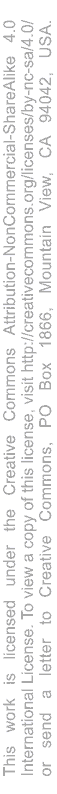# Volts, Amps, Ohms and Watts

Ohmn's Law - the relationship between basic electrical quantities.

## Basics

### Voltage

Sometimes called 'pressure' or 'potential difference', voltage is measured between two points and is the force which drives current through a conductor.

Voltage is measured in volts, V. In some circumstances it is represented by the letter E

### Current

Current is the quantity of electricity flowing in a circuit.

Current is measured in amps, A. In equations, current is usually given the letter I.

### Resistance

Resistance is the proeprty of a substance which determines how easy or difficult it is for electricity to pass through it.

Resistance is measured in ohms, Ω. In equations, resistance is usually given the letter R.

### Power

Power is the rate that electrical energy is transferred.

Power is measured in watts, W. In equations, power is usually given the letter P.

## Relationship

Given any two of the values, the other two can be calculated.

For a fixed resistance, increasing the voltage will increase the current. Decreasing the voltage decreases the current.

For a fixed voltage, increasing the resistance will decrease the current. Decreasing the resistance increases the current.

Power is the voltage multiplied by the current.